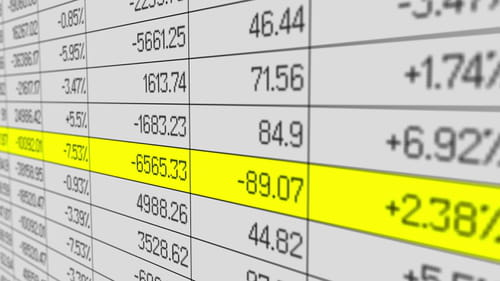1
Thanks

A few words of thanks would be greatly appreciated.

# How to Update Dates in Excel Spreadsheets

Do you use Excel spreadsheets to keep track of your weekly, monthly or annual expenses but find yourself updating all relevant date and content information manually? There is an easier, more effective solution and in this article we will provide you with a macro formula which will help with the automatic update of information.## Macro to Update Dates in Excel Spreadsheet

The macro will bring up an input box. there type the year for e.g. 2020 or 2021. You can format date column A as you like.

Test the macro and decide.

`Sub test()Dim y As Integer, d As Integery = InputBox("type the year, e.g. 2010")If y Mod 4 = 0 Thend = 366Elsed = 365End IfRange("a1") = "1/1/" & yRange(Range("A1"), Cells(d, "A")).DataSeries Rowcol:=xlColumns, _Type:=xlChronological, Date:= _        xlDay, Step:=1, Trend:=FalseRange("B1").Formula = "=weekday(A1)"Range("B1").AutoFill Range(Range("B1"), Cells(d, "B"))End Sub`

Should this macro not provide the solution to your problem, this article contains multiple macro solutions which might be of help.